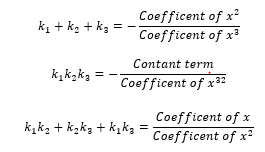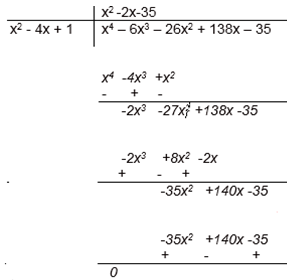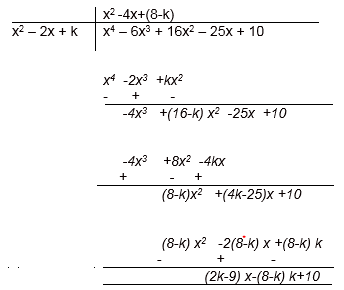# NCERT Solutions for Class 10 Maths Polynomials Exercise 2.4

## NCERT Solutions for Class 10 Maths Chapter 2 Exercise 2.4

In this page we have NCERT Solutions for Class 10 Maths Polynomials for EXERCISE 2.4 on pages 36 and 37 . Hope you like them and do not forget to like , social_share and comment at the end of the page.
If $k_1$, $k_2$, $k_3$ are the zeroes of the cubic polynomial $ax^3 + bx^2 + cx + d$, thenQuestion 1.
Verify that the numbers given alongside of the cubic polynomials below are their zeroes. Also verify the relationship between the zeroes and the coefficients in each case:
(i) 2x3+x2-5x+ 2; 1/2, 1, -2
(ii)x3- 4x2+5x -2; 2, 1, 1
(i)p(x) = 2x3+x2-5x+ 2
Now for verification of zeroes, putting the given value in x.
P (1/2) = 2(1/2)3+(1/2)2- 5(1/2)+ 2
=(2×1/8)+ 1/4 - 5/2+ 2
= 1/4+ 1/4 - 5/2+ 2
= 1/2 - 5/2+ 2 = 0
P (1) = 2(1)3+(1)2- 5(1)+ 2
=(2×1)+ 1 - 5 + 2
= 2+ 1 - 5 + 2 = 0
P (-2) = 2(-2)3+(-2)2- 5(-2)+ 2
=(2 × -8)+ 4 + 10+ 2
= -16 + 16= 0
Thus, 1/2, 1 and -2 are the zeroes of the given polynomial.
Comparing the given polynomial with ax3+bx2+cx+ d, we get a=2, b=1, c=-5, d=2
Also,k1=1/2, k2=1 and k3=-2Now,
-b/a = k1 + k2 +k3
⇒ 1/2 = 1/2+ 1 - 2
⇒ 1/2 = 1/2

c/a =k1k2+k2k3+k1k3
⇒ -5/2 = (1/2 × 1)+ (1 × -2)+ (-2 × 1/2)
⇒ -5/2 = 1/2 - 2 - 1
⇒ -5/2 = -5/2
-d/a = k1k2k3
⇒ -2/2 = (1/2 × 1 × -2)
⇒ -1 = 1
Thus, the relationship between zeroes and the coefficients are verified.
(ii) p(x) =x3- 4x2+5x -2
Now for verification of zeroes, putting the given value in x.
p(2) = 23- 4(2)2+ 5(2)- 2
= 8 - 16 +10 - 2
= 0
p(1) = 13- 4(1)2+5(1)- 2
= 1 - 4+5 - 2
= 0
p(1) = 13- 4(1)2+5(1)- 2
= 1 - 4+5 - 2
= 0
Thus, 2, 1 and 1 are the zeroes of the given polynomial.
Comparing the given polynomial with ax3+bx2+cx+ d, we get a=1, b=-4, c=5, d=-2
Also,k1=2, k2=1 and k3=1Now,
-b/a = k1 + k2 +k3
⇒ 4/1 = 2+ 1 + 1
⇒ 4 = 4

c/a =k1k2+k2k3+k1k3
⇒ 5/1 = (2 × 1)+ (1 × 1)+ (1 × 2)
⇒ 5 = 2 + 1+ 2
⇒ 5 = 5
-d/a = k1k2k3
⇒ 2/1 = (2 × 1 × 1)
⇒ 2 = 2
Thus, the relationship between zeroes and the coefficients are verified.

Question 2.
Find a cubic polynomial with the sum, sum of the product of its zeroes taken two at a time, and the product of its zeroes as 2, –7, –14 respectively.
Let the polynomial beax3+bx2+cx+ dand the zeroes be k1, k2 and k3Then, k1 + k2 +k3 = -(-2)/1 = 2 = -b/a
k1k2+k2k3+k1k3= -7 = -7/1 = c/a
k1k2k3 = -14 = -14/1 = -d/a
We can take any value of a and then find the values of b,c and d
Taking a = 1
b = -2, c = -7 and d = 14
So, one cubic polynomial which satisfy the given conditions will bex3- 2x2 -7x+ 14

Question 3.
If the zeroes of the polynomialx3– 3x2+x+ 1 are a–b, a, a+b, find a and b.
Since, (a - b), a, (a + b) are the zeroes of the polynomialx3– 3x2+x+ 1.Therefore, sum of the zeroes = (a - b) + a + (a + b) = -(-3)/1 = 3
⇒ 3a = 3 ⇒ a =1
∴ Sum of the products of is zeroes taken two at a time = a(a - b) + a(a + b) + (a + b) (a - b) =1/1 = 1
a2- ab + a2+ ab + a2- b2= 1
⇒ 3a2- b2=1
Putting the value of a,
⇒ 3(1)2- b2= 1
⇒ 3 - b2= 1
⇒ b2= 2
⇒ b = ±√2
Hence, a = 1 and b = ±√2

Question 4
If two zeroes of the polynomial x4– 6x3– 26x2+ 138x – 35 are 2±√3,find other zeroes.
2+√3 and 2-√3 are two zeroes of the polynomial p(x) = x4– 6x3– 26x2+ 138x – 35.
So, $(x- 2 + \sqrt 3)(x -2 - \sqrt 3)$ is a factor of the polynomial
Multiplying them
$x^2- 4x + 1$ is a factor of polynomial p(x)
Another Method to get this would be
Let x = 2±√3
So, x-2 = ±√3
On squaring, we get x2- 4x + 4 = 3,
⇒ x2- 4x + 1= 0
Now, dividing p(x) by x2- 4x + 1p(x) = x4- 6x3- 26x2+ 138x - 35
= (x2- 4x + 1) (x2- 2x - 35)
= (x2- 4x + 1) (x2- 7x + 5x - 35)
= (x2- 4x + 1) [x(x - 7) + 5 (x - 7)]
= (x2- 4x + 1) (x + 5) (x - 7)
So (x + 5) and (x - 7) are other factors of p(x).
Therefore
- 5 and 7 are other zeroes of the given polynomial.

Question 5.
If the polynomial x4– 6x3+ 16x2– 25x + 10 is divided by another polynomial x2– 2x + k, the remainder comes out to be x + a, find k and a.

On dividing x4– 6x3+ 16x2– 25x + 10 by x2– 2x + kRemainder = (2k - 9)x - (8 - k)k + 10
But the remainder is given as x+ a.
On comparing their coefficients,
2k - 9 = 1
⇒ k = 10
⇒ k = 5 and,
-(8-k)k +10 = a
⇒ a = -(8 - 5)5 + 10 =- 15 + 10 = -5
Hence, k = 5 and a = -5

## Summary

1. NCERT Solutions for Class 10 Maths Polynomials Exercise 2.4 has been prepared by Expert with utmost care. If you find any mistake.Please do provide feedback on mail. You can download the solutions as PDF in the below Link also
2. This chapter 2 has total 4 Exercise 2.1 ,2.2,2.3 and 2.4. This is the Fourth exercise in the chapter.You can explore previous exercise of this chapter by clicking the link belowGo back to Class 10 Main Page using below links

### Practice Question

Question 1 What is $1 - \sqrt {3}$ ?
A) Non terminating repeating
B) Non terminating non repeating
C) Terminating
D) None of the above
Question 2 The volume of the largest right circular cone that can be cut out from a cube of edge 4.2 cm is?
A) 19.4 cm3
B) 12 cm3
C) 78.6 cm3
D) 58.2 cm3
Question 3 The sum of the first three terms of an AP is 33. If the product of the first and the third term exceeds the second term by 29, the AP is ?
A) 2 ,21,11
B) 1,10,19
C) -1 ,8,17
D) 2 ,11,20